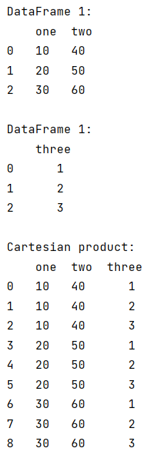# How to perform CROSS JOIN with pandas dataframe?

Given a Pandas DataFrame, we have to perform CROSS JOIN with it.
Submitted by Pranit Sharma, on August 07, 2022

Pandas is a special tool that allows us to perform complex manipulations of data effectively and efficiently. Inside pandas, we mostly deal with a dataset in the form of DataFrame. DataFrames are 2-dimensional data structures in pandas. DataFrames consist of rows, columns, and data.

And since a DataFrame consists of rows and column, it is easy to understand and analyse the data or we can say that pandas provides a slippery way to learn and to deliver a product in the form of an analysed data set.

Here, we'll learn to perform Cross Join in pandas DataFrame, Cross Join is similar to the cartesian product which is performed when we have data in form of rows and columns.

Cartesian product is a special product where each row value of one array is multiplied by each column of another array, but in pandas, each element from the row will be added to a new DataFrame column-wise.

We can perform cartesian product or Cross Join in pandas by using merge() method with a required parameter called 'how=cross'.

Let us understand with the help of an example,

## Python code to perform CROSS JOIN with pandas dataframe

```# Importing pandas package
import pandas as pd

# Creating a dictionary
d= {'one':[10,20,30],'two':[40,50,60]}

d2 = {'three':[1,2,3]}

# Creating two Dataframes
df1 = pd.DataFrame(d)

df2 = pd.DataFrame(d2)

# Display DataFrames
print("DataFrame 1:\n",df1,"\n")

print("DataFrame 1:\n",df2,"\n")

# Cartesian product
result = df1.merge(df2, how='cross')

# Display result
print("Cartesian product:\n",result)
```

Output: